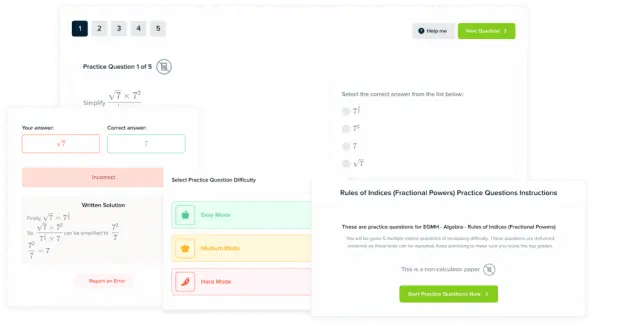# Gas Calculations Worksheets, Questions, and Revision

GCSEAQAChemistry Higher

## Gas Calculations

One of the most interesting properties of gasses is the volume of space that they occupy. All gasses, no matter the substances, will occupy the same volume of space per mole at a given temperature and pressure. At $20\degree \text{C}$ and $1\text{ atm (atmospheres)}$ of pressure, one mole of gas will occupy a volume $24\text{ dm}^3$. This will be true no matter what substance makes up the gas. This means that we can always calculate the moles of a gas (and therefore its mass) in a given volume provided we know it’s temperature and pressure.

## Calculating Moles and Volumes

Calculation of the moles or volume of a gas is relatively simple. If we know one, we are able to find the other thought the following relationship:

$\text{Volume of Gas at }20\degree \text{C}\text{ and }1\text{ atm}$$=$$24 \times \text{Moles of Gas Present}$

If we know the volume occupied by a gas at $20\degree \text{C}$ and $1\text{ atm}$ of pressure we can calculate the number of moles present by rearranging this formula.

It is important to remember that this relationship will only hold for gasses at $20\degree\text{C}$ and $1\text{ atm}$. At other temperatures or pressures, the volume occupied by one mole of gas will be different and so this different volume will need to be used in the above equation.

GCSEChemistry HigherAQA

## Calculating Gas Volumes from Equations

One result of this property of gasses is that, if we have a balanced equation where one or more of the chemicals involved is a gas, we can work out the volume of space occupied by this gas at $20\degree\text{C and }1\text{ atm}$. This because the balanced equation will tell us the ratios of moles of each chemical are present in the reaction

Take the example of the reaction between hydrochloric acid $\left(\text{HCl}\right)$ and sodium hydrogen carbonate $\left(\text{NaHCO}_3\right)$:

$\text{HCl}_{\text{ (aq)}}+\text{NaHCO}_{3\text{ (s)}} \rarr \text{NaCl}_{\text{ (s)}}+\text{H}_2\text{O}_{\text{ (l)}}+\text{CO}_{2\text{ (g)}}$

This equation tells us that the ratio of moles of $\left(\text{HCl}\right)$ & $\left(\text{NaHCO}_3\right)$ to $\text{CO}_2$ is $1:1$. As such, if we know the mass of either $\left(\text{HCl}\right)$or $\left(\text{NaHCO}_3\right)$, we can calculate the volume of $\text{CO}_2$ evolved.

GCSEChemistry HigherAQA

## Example 1: Calculating Volume

Calculate the volume occupied by $\textcolor{#00bfa8}{2.3\text{ moles}}$ of nitrogen gas at $20\degree\text{C and }1\text{ atm}$:

[1 mark]

Volume of Nitrogen at $20\degree \text{C}$ and $1\text{ atm}$:

$= 24 \times \text{Moles of Nitrogen Present}\\ = 24\times \textcolor{#00bfa8}{2.3}\\ =\textcolor{#008d65}{55.2\text{ dm}^3}$

GCSEChemistry HigherAQA

## Example 2: Calculating Moles

Calculate the moles of oxygen present in $\textcolor{#00bfa8}{179\text{ dm}^3}$ of gas at $20\degree\text{C and }1\text{ atm}$:

[1 mark]

Volume of Oxygen at $20\degree \text{C}$ and $1\text{ atm}$:

$= 24 \times \text{Moles of Oxygen Present}$

$\text{Moles of Oxygen} =$$\frac{\text{Volume of Oxygen}}{24}\\ \, \\= \frac{\textcolor{#00bfa8}{179}}{24}\\ \, \\ =\textcolor{#008d65}{7.5\text{ mol}}$

GCSEChemistry HigherAQA

## Example 3: Calculating Volumes from Equations

Calculate the volume of $\text{CO}_2$ released when $\textcolor{#00bfa8}{8.35\text{ g}}$ of $\text{NaHCO}_3$ $(\text{M}_r =\textcolor{#f21cc2}{84})$ reacts with $\text{HCl}$ at $20\degree\text{C and }1\text{ atm}$:

[3 marks]

Step 1: calculate the moles of $\text{NaHCO}_3$ that have reacted:

$\text{Moles NaHCO}_3 = \frac{\text{Mass NaHCO}_3}{\text{M}_r \text{NaHCO}_3}\\ \, \\ =\frac{\textcolor{#00bfa8}{8.35}}{\textcolor{#f21cc2}{84}}\\ \, \\=\textcolor{#008d65}{0.099\text{ mol}}$

Step 2: calculate the moles of $\text{CO}_2$ that are evolved from the balanced equation of the reaction:

$\text{HCl}_{\text{ (aq)}}+\text{NaHCO}_{3\text{ (s)}} \rarr \text{NaCl}_{\text{ (s)}}+\text{H}_2\text{O}_{\text{ (l)}}+\text{CO}_{2\text{ (g)}}$

$\text{Ratio of Moles}=1:1$

$\text{Moles of CO}_2=\text{Moles of NaHCO}_3$

Step 3: calculate the volume occupied by $\text{CO}_2$ at $1\degree\text{C and }1\text{ atm}$:

Volume of $\text{CO}_2$ at $20\degree \text{C}$ and $1\text{ atm}$:

$= 24 \times \text{Moles of CO}_2\\ \, \\= 24\times 0.099\\ \, \\=\textcolor{#008d65}{2.4\text{ dm}^3}$

GCSEChemistry HigherAQA

## Gas Calculations Worksheets, Questions, and Revision Example Questions

\begin{aligned}\text{Volume of HCl at }20\degree \text{C}\text{ and }1\text{ atm} &= 24 \times \text{Moles of HCl Present}\\ &=24 \times 0.070 \\ &=\underline{1.68\text{ dm}^3}\end{aligned}Gold Standard Education

Firstly, convert the volume of the room into $\text{ dm}^3$:

\begin{aligned}\text{Volume in dm}^3&=\frac{\text{Volume in cm}^3}{1000}\\ &=\frac{70000000}{1000}\\ &=\underline{70000\text{ dm}^3}\end{aligned}

Second, calculate the moles of air present:

\begin{aligned}\text{Moles of Air}&=\frac{\text{Volume of Room}}{24}\\ &=\frac{70000}{24}\\ &=\underline{2917\text{ mol}}\end{aligned}Gold Standard Education

Calculation should be broken down into steps. One mark per correct step.

Balanced Equation:

$\text{HCl}_{\text{ (aq)}}+\text{NaHCO}_{3\text{ (s)}} \rarr \text{NaCl}_{\text{ (s)}}+\text{H}_2\text{O}_{\text{ (l)}}+\text{CO}_{2\text{ (g)}}$

Step 1: Calculate moles of $\text{NaHCO}_3$.

\begin{aligned}\text{Moles NaHCO}_3 &= \frac{\text{Mass NaHCO}_3}{\text{M}_r \text{NaHCO}_3}\\ &=\frac{4.67}{84}\\ &=\underline{0.056\text{ mol}}\end{aligned}

Step 2: Calculate Moles of $\text{CO}_2$.

$\text{Ratio of Moles}=1:1$

$\text{Moles of CO}_2=\text{Moles of NaHCO}_3$

Step 3: Calculate the volume of $\text{CO}_2$$\text{ at } 20\degree\text{C and }1\text{ atm}$:

\begin{aligned}\text{Volume of CO}_2\text{ at }20\degree \text{C}\text{ and }1\text{ atm} &= 24 \times \text{Moles of CO}_2\\ & 24\times 0.056\\ &=\underline{1.34\text{ dm}^3}\end{aligned}Gold Standard Education

## Gas Calculations Worksheets, Questions, and Revision Worksheet and Example Questions

### Yield and atom economy of chemical reactions. Questions

GCSEOfficial MMEProduct